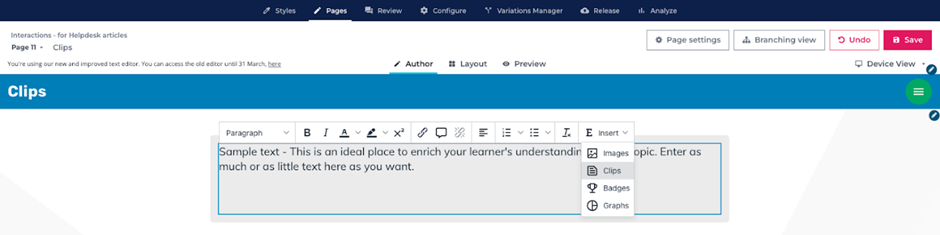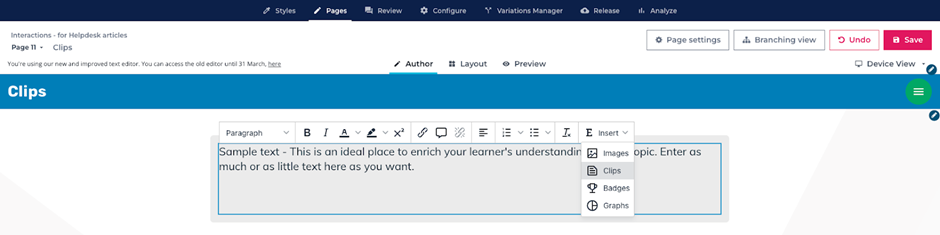# Using Clips to display a learner’s score

A useful way to use Clips when assessing learners is to display the learner’s score to them on a results page to show how well they have done. By using Clips, you can play back a learner’s score for a given Chapter, or the total score for the entire course.

Clips can be embedded directly into text so you can seamlessly integrate them into your course's content.

## Displaying a learner’s total score for the course

1. Create a text box on the page where you’d like to display your learner’s score and select Insert Clips:2. From the popup window, there are two options that can display the current total score:

• Total score (%) which let learners know the percentage of questions they answered correctly within the course. In Author mode this will appear as`{total.score}`
•Total score (Number) which will display the number of points achieved in the Chapter based on the Score Weighting of each page in the Chapter. In Author mode this will appear as`{total.score.raw}`

Here's an example of how each Clip will look to the learner.

In a course with 4 scored pages, each of which with a Score Weighting of 50, the learner has answered 3 out 4 questions correctly.

Total score (%) will show the score as a percentage value which in this case would be 75%.

Total score (Number) will show the score in its raw value which in this case would be 150 (three questions worth 50 points each = 150)

## Displaying a learner’s score for the current chapter

1. Create a textbox on the page where you’d like to display your learner’s score for the current chapter, and select Insert Clips:2. From the popup window, there are two options that can display the current chapter score:

• Current chapter score (%) which will let learners know the percentage of questions they answered within the Chapter. In Author mode this will appear as`{total.chapter.score}`
• Current chapter score (Number) which will display the number of points achieved in the Chapter based on the Score Weighting of each page in the Chapter. In Author mode this will appear as`{total.chapter.score.raw}`

Here's an example of how this will look to the learner.

I have two chapters in my course, both of which have two scored questions each, all weighted at 50. A learner answers 1 out 2 questions correctly in Chapter 1 but both questions correctly in Chapter 2.

A Current chapter score (%) Clip placed in Chapter 2 will show the score achieved only for questions in that chapter. Since the learner answered them all correctly in Chapter 2, this Clip would show 100%.

A Current chapter score (Number) Clip placed in Chapter 2 would show 100 (two questions worth 50 points each = 100).